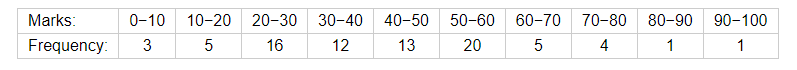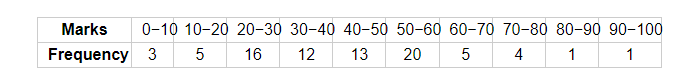# The marks in science of 80 students of class X are given below:

Question:

The marks in science of 80 students of class X are given below: Find the mode of the marks obtained by the students in science.Solution:Here, the maximum frequency is 20 so the modal class is 50−60.

Therefore,

$l=50$

$h=10$

$f=20$

$f_{1}=13$

$f_{2}=5$

Now,

Mode $=l+\frac{f-f_{1}}{2 f-f_{1}-f_{2}} \times h$

$=50+\frac{20-13}{40-13-5} \times 10$

$=50+\frac{7}{22} \times 10$

$=50+\frac{70}{22}$

$=50+3.17$

Thus, the mode of the marks obtained by the students in science is 53.17.